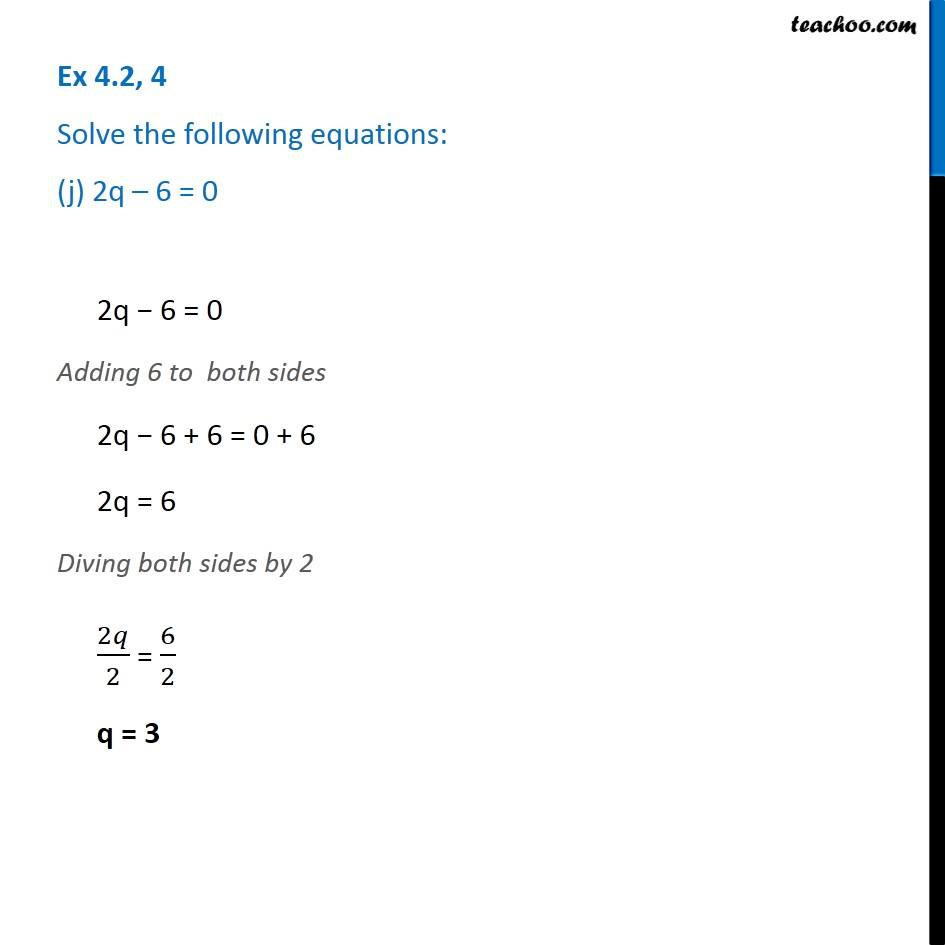1. Chapter 4 Class 7 Simple Equations
2. Serial order wise
3. Ex 4.2

Transcript

Ex 4.2, 4 Solve the following equations: (j) 2q – 6 = 02q − 6 = 0 Adding 6 to both sides 2q − 6 + 6 = 0 + 6 2q = 6 Diving both sides by 2 2𝑞/2 = 6/2 q = 3

Ex 4.2

Chapter 4 Class 7 Simple Equations
Serial order wise